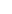It weighs every closing price (not necessarily) depending on how recent it is, giving more importance to recent prices and less importance on past prices. To calculate it we add the closing values multiplied by their weight and divide the result by the sum of the weights. To calculate e.g. the 6-days WMA closing price of a coin, we follow these steps:

1. We have the last 6 closing prices ​​so that the oldest (5 days ago) has the number 1, the penultimate the number 2... and the most recent the number 6. The above numbers (1, 2, 3, 4, 5, 6) are our weights.

2. Using the above weights we multiply the closing price of 5 days ago with 1, the closing price of 4 days go with 2 ... and the last closing price with 6.

3. We add the products of step 2.

4. We add the weights: 1 + 2 + 3 + 4 + 5 + 6 = 21.

5. We divide the result of step 3 with the result of step 4. The result gives us the WMA’s value.

WMA calculation example:

Suppose we are given the closing prices of the last 6 days:

 Day 6 5 4 3 2 Today Closing price 5 3 2 4 7 9

To calculate the WMA of the last 6 days, we follow the 5 steps we described earlier:

1. The weights are: 1,2,3,4,5,6

2. We multiply the closing values by their weights: 5 * 1 = 5, 3 * 2 = 6, 2 * 3 = 6, 4 * 4 = 16, 7 * 5 = 35 and 9 * 6 = 54

3. We add the products from step 2: 5 + 6 + 6 + 16 + 35 + 54 = 122

4. We add the weights: 1 + 2 + 3 + 4 + 5 + 6 = 21

5. We divide the result of step 3 with the result of step 4: 122/21 = 5.81to signal pageWeighted Moving Average (WMA) ❺❺❺ - average rating 5 from 5 (based on 193 user reviews)